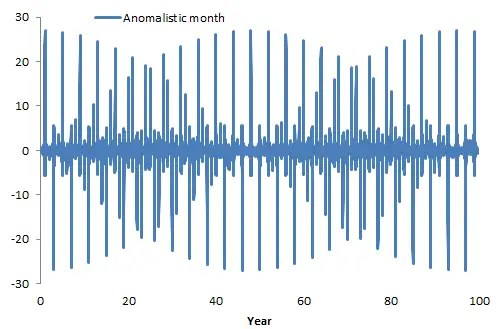# Biennial Connection to Seasonal Aliasing

[mathjax]I found an interesting mathematical simplification relating seasonal aliasing of short-period cycles with a biennial signal.

If we start with a signal of an arbitrary frequency $$omega_L = 2pi/T_L$$$L(t) = k cdot sin(omega_L t + phi)$

and then modulate it with a delta function array of one spike per year$s(t) = sumlimits_{i=1}^n a_i sin(2 pi t i +theta_i)$

This is enough to create a new aliased cycle that is simply the original frequency $$omega_L$$ summed with an infinite series of that frequency shifted by multiples of $$2pi$$.$f(t) = k/2 sumlimits_{i=1}^n a_i sin((omega_L - 2 pi i)t +psi_i) + ...$

This was derived in a previous post. So as a concrete example, the following figure is a summed series of f(t) — specifically what we would theoretically see for an anomalistic lunar month cycle of 27.5545 days aliased against a yearly delta.Fig 1: Series expansion of aliasing

If you count the number of cycles in the span of 100 years, it comes out to a little less than 26 cycles, or an approximately 3.9 year aliased period. If a low-pass filter is applied to this time series, which is likely what would happen in the lagged real world, a sinusoid of period 3.9 years would emerge.

The interesting simplification is that the series above can also be expressed exactly as a biennial + odd-harmonic expansion$[cos(pi t) + cos(3 pi t) + cos(5 pi t) + ...] cdot sin(frac{2pi}{T} t)$

where T is given by$1/T = 1/2 + int(1/T_L) - 1/T_L$

where the function “int” truncates to the integer part of the period reciprocal. Since $$T_L$$ is shorter than the yearly period of 1, then the reciprocal is guaranteed to be greater than one.

As a check, if we take only the first biennial term of this representation (ignoring the higher frequency harmonics) we essentially recover the same time series.Fig 2: Biennial modulation term

What is interesting about this factoring is that a biennial modulation may naturally emerge as a result of yearly aliasing, which is possibly related to what we are seeing with the ENSO model in its biennial mode. Independent of how many lunar gravitational terms are involved, the biennial modulation would remain as an invariant multiplicative factor.

For the ENSO signal, an anomalistic term corresponding to a biennial modulation operating on a 4.085 year sinusoid appears significant in the model

1/4.085 = 1/2 + int(365.242/27.5545) – 365.242/27.5545

which is expanded as this pair of biennially split factors (see ingredient #5 in the ENSO model)

1/4.085 ~ 2/3.91 – 2/1.34

## 7 thoughts on “Biennial Connection to Seasonal Aliasing”

1.Ian Wilson

Paul,

A. I think you may have a typo here:

1/4.085 ~ 2/3.91 – 2/1.34 this should read
1/4.085 ~ 2/3.91 – 2/7.52667

B. Given an anomalistic month = 27.554550 days (J2000)
the nearest whole harmonic of the one tropical year is:
365.242189/13 = 28.095553 days

then
(28.095553 x 27.554550) / (28.095553 – 27.554550) = 1430.9723235 days
__________________________________________= 3.917872 trop. years

and the nearest whole harmonic of two tropical years is:
2 x 365.242189 / 27 = 27.05497696 days

then
(27.05497696 x 27.554550) / (27.554550 – 27.05497696) = 1492.2496926 days
______________________________________________= 4.085644 trop. years

C. One suggestion that might help with your study.

You are using forcing terms with periods that alias the lunar anomalistic and draconic months with 1.0 and 2.0 tropical years. The problem with this approach is that (to second order) the Sun also plays a role in the determining the strength of the lunar tides.

This means that you need to deal with times where the lunar synodic month and anomalistic (or Draconic) month align and ask yourself how they realign with the tropical or sidereal year.

For example, the alignment between the synodic and anomalistic months takes place at multiples and half multiples of the Full Moon Cycle (FMC):

(29.5305889 x 27.554550) / (29.5305889 – 27.554550) = 411.78444836 days
____________________________________________= 1.21742849 trop. yrs.
____________________________________________= 1.2738474 sider. yrs.

These are just the Perigean Spring tides which take place every 205.9 days (= half of one FMC of 411.78445 days).

Simple maths shows you that the nearest multiple of the FMC (in sidereal years) that takes place nearest to 2.00 sidereal years is:

2 x 1.2738474 = 2.2547695 sidereal years which re-aligns with 2.00 sidereal years at a multiple of:

(2.2547695 x 2.00) / (2.2547695 – 2.00) = 17.700468 sidereal years

such that 5.0 x 17.700468 = 88.5023 sidereal years.

This is just the Gleissberg lunar tidal cycle.

Like

2.admin

A) It’s just sign reversed. This is irrelevant for a sinusoid

-1/4.085 ~ 2/3.91 – 2/1.34

B) That’s good to know

C) “The problem with this approach is that (to second order) the Sun also plays a role in the determining the strength of the lunar tides.”
I can marginally improve the fit by adding a 1.2181 year cycle, but that is 444.64 days and not 411 days. The latter contributes nothing significant.

You are right that this would be a second-order effect. I am not sure about including second-order effects at this point. It will degrade the information criteria score because of increased complexity. Perhaps some time in the future. For example, full-blown tidal analysis does include lots of extra factors, but that approach has solidified over the years, while this is still at the embryonic stage.

Like

3.admin

Reid, George C. “Seasonal and interannual temperature variations in the tropical stratosphere.” Journal of Geophysical Research: Atmospheres 99.D9 (1994): 18923-18932.

This is from one of the founders of the NOAA environmental science lab.

Like